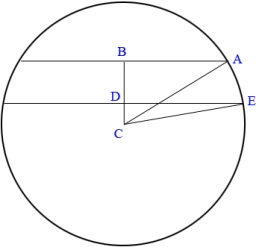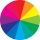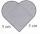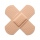# Area to perimeter

Calculate circle circumference if its area is 254.34cm2

o =  56.5343 cm

### Step-by-step explanation:Did you find an error or inaccuracy? Feel free to write us. Thank you!#### You need to know the following knowledge to solve this word math problem:

We encourage you to watch this tutorial video on this math problem:

## Related math problems and questions:

• Four circles1) Calculate the circle radius if its area is 400 cm square 2) Calculate the radius of the circle whose circumference is 400 cm. 3) Calculate circle circumference if its area is 400 cm square 4) Calculate the circle's area if perimeter 400 cm.
• Perimeter of the circleCalculate the perimeter of the circle in dm, whose radius equals the side of the square containing 0.49 dm2?
• Quarter of a circleCalculate the circumference of a quarter circle if its content is S = 314 cm2.
• CircleHow big is an area of a circle if its circumference is 51.2 cm?
• Area of a circleThe circumference of the circle is 150.72 centimeters. Calculate the area of the circle.
• The circumferenceThe circumference and width of the rectangle are in a ratio of 5: 1. its area is 216cm2. What is its length?
• Square s3Calculate the diagonal of the square, where its area is 0.49 cm square. And also calculate its circumference.
• Ratio of sidesCalculate the area of a circle with the same circumference as the circumference of the rectangle inscribed with a circle with a radius of r 9 cm so that its sides are in ratio 2 to 7.
• Circle - simpleCalculate the area of a circle in dm2 if its circumference is 31.4 cm.
• Math heartStylized heart shape created from a square with side 5 cm and two semicircles over his sides. Calculate the content area and its circumference.
• Two patchesPeter taped the wound with two rectangular patches (one over the other to form the letter X). The area sealed with both patches at the same time had a content of 40cm2 and a circumference of 30cm. One of the patches was 8cm wide. What was the width of the
• RectangleThe length of the rectangle are in the ratio 5:12 and the circumference is 238 cm. Calculate the length of the diagonal and area of rectangle.
• Rectangular trapeziumCalculate the perimeter of a rectangular trapezium when its content area is 576 cm2 and sice a (base) is 30 cm, height 24 cm.
• ShapePlane shape has a maximum area 677 mm2. Calculate its perimeter if perimeter is the smallest possible.
• Circle and rectangleA rectangle with sides of 11.7 cm and 175 mm is described by circle. What is its length? Calculate the content area of the circle described by this circle.
• RectangleThe length of the rectangle is 12 cm greater than 3 times its width. What dimensions and area this rectangle has if ts circumference is 104 cm.
• TerezaThe cube has an area of base 256 mm2. Calculate the edge length, volume, and area of its surface.